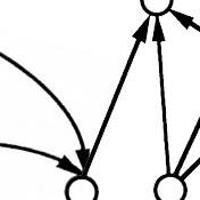## Linear separability

Linear separability is an important concept in neural networks. The idea is to check if you can separate points in an n-dimensional space using only n-1 dimensions. Lost it? Here's a simpler explanation.

## One Dimension

Lets say you're on a number line. You take any two numbers. Now, there are two possibilities:

1. You choose two different numbers
2. You choose the same number

If you choose two different numbers, you can always find another number between them. This number "separates" the two numbers you chose.

So, you say that these two numbers are "linearly separable".

But, if both numbers are the same, you simply cannot separate them. They're the same. So, they're "linearly inseparable". (Not just linearly, they're aren't separable at all. You cannot separate something from itself)

## Two Dimensions

On extending this idea to two dimensions, some more possibilities come into existence. Consider the following:

Here, we're like to seperate the point (1,1) from the other points. You can see that there exists a line that does this. In fact, there exist infinite such lines. So, these two "classes" of points are linearly separable. The first class consists of the point (1,1) and the other class has (0,1), (1,0) and (0,0).

Now consider this:

In this case, you just cannot use one single line to separate the two classes (one containing the black points and one containing the red points). So, they are linearly inseparable.

## Three dimensions

Extending the above example to three dimensions. You need a plane for separating the two classes.

The dashed plane separates the red point from the other blue points. So its linearly separable. If bottom right point on the opposite side was red too, it would become linearly inseparable .

## Extending to n dimensions

Things go up to a lot of dimensions in neural networks. So to separate classes in n-dimensions, you need an n-1 dimensional "hyperplane".

## Summary

So hopefully, you've understood how linear separability works. You'll be seeing this again and again in several other articles related to neural networks.

#### Related posts#### 78xx voltage regulator#### 7 unique neural network architectures#### Community Core Vision: An app for simple vision stuff

Utkarsh Sinha created AI Shack in 2010 and has since been working on computer vision and related fields. He is currently at Microsoft working on computer vision.